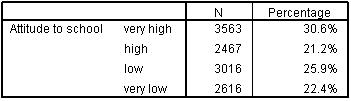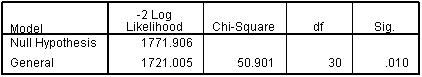# Using Statistical Regression Methods in Education Research# Ordinal Regression Quiz

Your total score is :0 / 16

Here is a fresh set of 16 questions for you to ponder… Click the relevant box or drag and drop the answers as appropriate! Click VIEW FEEDBACK when you’re ready to see the answers. When you want to move on just click EXIT QUIZ. Enjoy!
1. The bar charts below represent four different variables. Which of them may be suitable as an outcome variable for ordinal regression?2. Match the regression technique to the appropriate type of outcome variable:
 Regression technique Continuous Ordinal Binary Logistic Regression Ordinal Regression Multiple Linear Regression

4. The assumption of proportional odds states that:

5. The table below describes an ordinal outcome variable, attitude to school.Are the following statements about this data true or false? Please drag and drop each statement into the correct box. You may need a calculator!
 Statements True False The odds of being in the 'high' group or above (i.e. high or very high) are 1.07 More than 50% of the sample have a 'high' or 'very high' score. The odds of being in the 'low' group or above are 2.0 The odds of being in the 'very high' group are 0.31 The odds of being in the 'very low' group are 0.29

9. The table below provides the parameter estimates for an ordinal regression model. The outcome variable is ‘Attitude to school’, which ranges on a scale of 1 to 4, with lower values representing a more positive attitude to school. The explanatory variables are SEC, ethnicity and gender.Which of the following groups, when compared to the reference group for the relevant variable, contribute to the model to a statistically significant degree.

10. In the table for the previous question, what do the values in the ‘Estimate’ column represent?

11. Based on the same parameter estimates table, which of the following conclusions are supported by the results in relation to gender? You may choose more than one answer.

12. Based on the same parameter estimates table, what are the odds that an Indian student will have a higher score on the attitude to school measure relative to a white british student?

13. This table shows the Pseudo R-square statistics for the model we have discussed in the previous questions:The model describes a large amount of the variance in student attitude to school. True or false?

14. The table below shows the ‘Test of Parallel Lines’ output for an ordinal regression:Has the assumption of proportional odds been met?

15. What should be done if the proportional odds assumption is violated during an ordinal regression? You may choose more than one answer.

16. It could be argued that it is easier to split ordinal outcome variables down in to multiple binary variables and model the data using a series of logistic regression analyses rather than a single ordinal regression analysis. Which of the following statements are true (please tick all that apply)?

Privacy statement
This quiz is anonymous. No data which personally identifies you is collected on the quiz, and the data you provide is used solely to help us improve the delivery of our courses.

Page contact: Feedback to ReStore team Last revised: Thu 19 May 2011